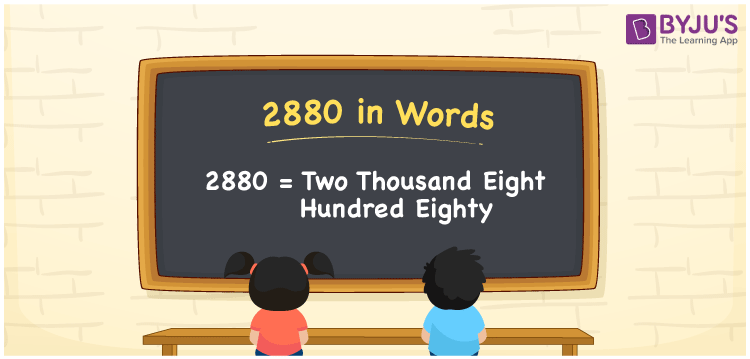# 2880 in Words

2880 in words is written as Two thousand eight hundred eighty. In both the International System of Numerals and the Indian System of Numerals, 2880 in words, is written as Two thousand eight hundred eighty. The number 2880 is a Cardinal Number as it represents some quantity. For example, “the dress costs 2880 rupees”.

 2880 in Words Two thousand eight hundred eighty Two thousand eight hundred eighty in Number 2880

## 2880 in English Words

2880 in English words is read as “Two thousand eight hundred eighty”.## How to Write 2880 in Words?

To write 2880 in words, we shall use the place value chart. In the place value chart, put 2 in the thousands, 8 in the hundreds and tens, and 0 in the ones, respectively. Let us make a place value chart to write the number 2880 in words.

 Thousands Hundreds Tens Ones 2 8 8 0

Thus, we can write the expanded form as

2 × Thousand + 8 × Hundred + 8 × Ten + 0 × One

= 2 × 1000 + 8 × 100 + 8 × 10 + 0 × 1

= 2000 + 800 + 80 + 0

= 2880

= Two thousand eight hundred eighty.

2880 is a natural number which is the successor of 2879 and the predecessor of 2881.

2880 in words – Two thousand eight hundred eighty

• Is 2880 an odd number? – No
• Is 2880 an even number? – Yes
• Is 2880 a perfect square number? – No
• Is 2880 a perfect cube number? – No
• Is 2880 a prime number? – No
• Is 2880 a composite number? – Yes

## Frequently Asked Questions on 2880 in Words

Q1

### How to write 2880 in words?

2880 in words is written as Two thousand eight hundred eighty.
Q2

### How to write 2880 in words in the International and Indian System of Numerals?

In both, the system of numerals, 2880 in words, is written as Two thousand eight hundred eighty.
Q3

### How to write 2880 in a place value chart?

In the place value chart, write 2 in the thousands, 8 in the hundreds and tens, and 0 in the ones, respectively.